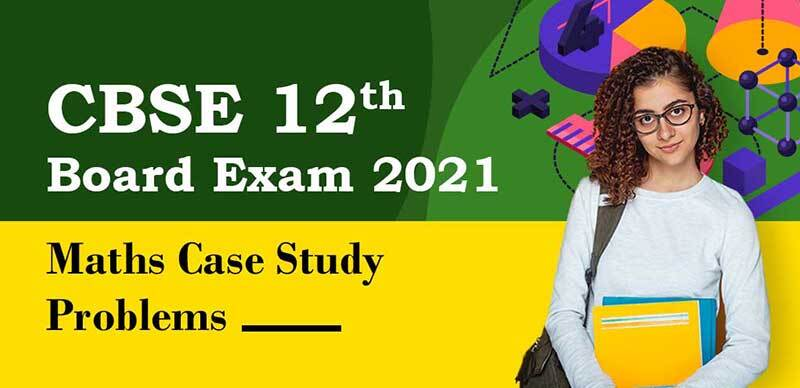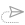# CBSE 12th Exam 2021 : Maths Case Study Problems

In Coming Exams, CBSE will ask two Case Study Questions in the CBSE class 12 maths questions paper. Each theme will have five questions and students will have a choice to attempt any four of them.

Here are some example questions Based On Case Study Problems:

Question-1

Read the case study carefully and answer any four out of the following questions:

Three friends Ganesh, Dinesh, and Ramesh went for playing a Tug of war game. Team A, B, and C belong to Ganesh, Dinesh, and Ramesh respectively.
Teams A, B, C have attached a rope to a metal ring and are trying to pull the ring into their own area (team areas shown below).
Team A pulls with F1 = 4i + 0j KN
Team B → F2 = -2i+ 4j  KN
Team C → F3 = -3i - 3j KN1. Which team will win the game?
1. Team B
2. Team A
3. Team C
4. No one
2. What is the magnitude of the team's combine Force?
1. 7 KN
2. 1.4 KN
3. 1.5 KN
4. 2 KN
3. In with direction approx the ring getting pulls:
1. 2.0 radian
2. 2.5 radian
3. 2.4 radian
4. 3 radian
4. What is the magnitude of the force of Team B?
1. 25–√25 KN
2. 6 KN
3. 2 KN
4. 6–√6 KN
5. How many KN Force is applied by Team A?
1. 5 KN
2. 4 KN
3. 2 KN
4. 16 KN

Answer Key:

1. (a) Team B
2. (b) 1.4 KN
3. (c) 2.4 radian
4. (a) 25–√25 KN
5. (b) 4 KN

Question-2

Read the case study carefully and answer any four out of the following questions:

Three shopkeepers Ram Lal, Shyam Lal, and Ghansham are using polythene bags, handmade bags (prepared by prisoners), and newspaper's envelope as carry bags. It is found that the shopkeepers Ram Lal, Shyam Lal, and Ghansham are using (20,30,40), (30,40,20), and (40,20,30) polythene bags, handmade bags, and newspapers envelopes respectively. The shopkeeper's Ram Lal, Shyam Lal, and Ghansham spent ₹250, ₹270, and ₹200 on these carry bags respectively.

1.  What is the cost of one polythene bag?
1. ₹ 1
2. ₹ 2
3. ₹ 3
4. ₹ 5
2. What is the cost of one handmade bag?
1. ₹ 1
2. ₹ 2
3. ₹ 3
4. ₹ 5
3. What is the cost of one newspaper bag?
1. ₹ 1
2. ₹ 2
3. ₹ 3
4. ₹ 5
4. Keeping in mind the social conditions, which shopkeeper is better?
1. Ram Lal
2. Shyam Lal
3. Ghansham
4. None of these
5. Keeping in mind the environmental conditions, which shopkeeper is better?
1. Ram Lal
2. Shyam Lal
3. Ghansham
4. None of these

Answer Key:

1. (a) ₹1
2. (b) ₹ 2
3. (d) ₹ 5
4. (b) Shyam Lal
5. (a) Ram Lal

Question-3

Read the case study carefully and answer any four out of the following questions:

Three friends Ravi, Raju, and Rohit were doing buying and selling stationery items in a market. The price per dozen of Pen, Notebook, and toys are Rupees x, y, and z respectively.
Ravi purchases 4 dozens of notebooks and sells 2 dozens of pens and 5 dozens of toys. Raju purchases 2 dozens of toys and sells 3 dozens of pens and 1 dozen of notebooks. Rohit purchases one dozen of pens and sells 3 dozens of notebooks and one dozen toys. In the process, Ravi, Raju, and Rohit earn ₹ 1500, ₹ 100, and ₹400 respectively.Answer the following questions using the matrix method:

1. What is the price of one dozen of pens?
1. ₹ 100
2. ₹ 200
3. ₹ 300
4. ₹ 400
2. What is the total price of one dozen of pens and one dozen of notebooks?
1. ₹ 100
2. ₹ 200
3. ₹ 300
4. ₹ 400
3. What is the sale amount of Ravi?
1. ₹ 1000
2. ₹ 1100
3. ₹ 1300
4. ₹ 1200
4. What is the amount of purchases made by all three friends?
1. ₹ 1200
2. ₹ 1500
3. ₹ 1300
4. ₹ 1400
5. What is the price of sales made by all three friends?
1. ₹ 3000
2. ₹ 2500
3. ₹ 2700
4. ₹ 2400

Answer Key:

1. (a) ₹ 100
2. (c) ₹ 300
3. (d) ₹ 1200
4. (b) ₹ 1500
5. (c) ₹ 2700

Question-4

Read the case study carefully and answer any four out of the following questions:

Dinesh is having a jewelry shop at Green Park, Normally he does not sit in the shop as he remains busy in political meetings. The manager Lisa takes care of jewelry shop where she sells earrings and necklaces. She sells earrings for ₹ 30 and necklaces for 40. It takes 30 minutes to make a pair of earrings and 1 hour to make a necklace, and there are 10 hours a week to make jewelry. In addition, there are only enough materials to make 15 total jewelry items per week. The profit of 15 and 20 is made on each pair of earrings and a necklace respectively.

The graph of the equations formed is shown here:Answer the following questions:

1. What is the first inequality formed?
1. 0.5x + y ≤ 10
2. x +0.5 y ≤ 10
3. 0.5x + y ≤ 15
4. x + 0.5 y ≤ 20
2. What is the second inequality formed?
1. 0.5x + y ≤ 10
2. x + y ≤ 10
3. x + y ≤ 15
4. x + 0.5 y ≤ 10
3. In order to maximize the profits, per week how many pair of earrings and necklaces should be made?
1. 10 and 5
2. 5 and 10
3. 15 and 5
4. 5 and 15
4. What would be the profit if 5 pairs of earrings and 5 necklaces are made?
1. 150
2. 300
3. 175
4. 250
5. What would be the maximum possible profit?
1. 150
2. 300
3. 175
4. 250

Answer Key:

1. 0.5x + y  ≤ 10
2. x + y ≤ 15
3. 10 and 5
4. 175
5. 250

Question-5

Read the case study carefully and answer any four out of the following questions:

A telephone company in a town has 500 subscribers on its list and collects fixed charges of 300 per subscriber per year. The company proposes to increases the annual subscription and it is believed that for every increase of 1 one subscriber will discontinue the service.1. If x be the annual subscription  then the total revenue of the company after increment will be:
1. R(x) = -x2 + 200x + 150000
2. R(x) = x2 - 200x - 140000
3. R(x) = 200x2 + x + 150000
4. R(x) = -x2 + 100 x + 100000
2. To find maximum profit we put
1. R'(x) = 0
2. R'(x) > 0
3. R'(x) < 0
4. R''(x) = 0
3. How much fee the company should increase to have maximum profit?
1. Rs. 150
2. Rs. 100
3. Rs. 200
4. Rs. 250
4. Find the maximum profit that the company can make if the profit function is given by P(x) = 41 + 24x - 18x2.
1. 25
2. 44
3. 45
4. 49
5. Find both the maximum and minimum value respectively of 3x4 - 8x3 + 48x + 1 on the interval [1, 4].
1. -63, 257
2. 258, -63
3. 257, -63
4. -63, -257

Answer Key:

1. (a) -x+ 200x + 150000
2. (a) R'(x) = 0
3. (b) Rs.100
4. (d) 49
5. (c) 257, -63

#### updates##### NTA NEET 2021 : Biology Chapter-Wise Speed Tests With Solution

21 April, 2021, 5:22 pm##### CBSE Class 12th - CHEMISTRY : Chapterwise Case Study Question & Solution

21 April, 2021, 12:18 pm##### CBSE Class 12th - PHYSICS : Chapterwise Case Study Question & Solution

20 April, 2021, 4:00 pm##### NEET Crash Course 2021 : DAY 6 - Quick Revision, DPPs, PYQ & Assignments

20 April, 2021, 1:58 pm##### ICSE/ISC Board Exam 2021 : 10th Exam Cancelled, 12th Exam Postponed, Check Here

20 April, 2021, 10:41 am##### CBSE 12th 2021 : English Core Sample Question Paper with Solution 2021

20 April, 2021, 9:15 amWe are providing something unique, useful and most importantly fun. By giving students a tool to find instant solutions to their doubts, we’re trying to make every student self-sufficient in practicing & completing their homework

### Follow Us

Copyright ©2021 All Rights Reserved, selfstudys.com